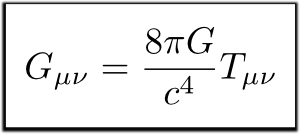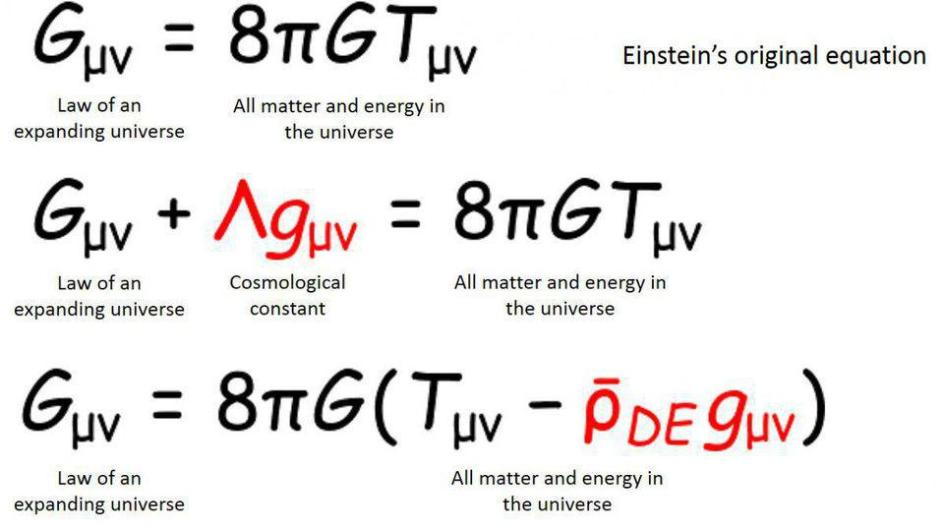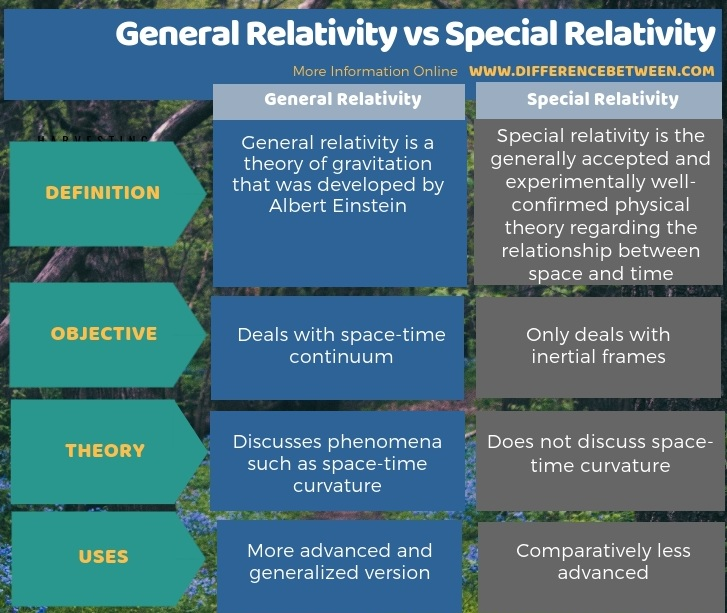# General Theory Of Relativity Study Guide

INTRODUCTION

The general theory of relativity was first developed by Albert Einstein. This theory is the latest description of modern physics. This theory explains gravity as the distortion of space curvature due to the effect of matter or energy. The theory of general relativity also has some consequences, such as gravitational time dilation and bending of light rays.

## DEFINITION OF GENERAL THEORY OF RELATIVITY

• The general theory of relativity is defined as the most towering observation of 20th-century physics.
• Albert Einstein published this theory in 1916.
• This theory mainly states that time and space both are relative, and so all the motion must also be relative to the frame of reference.
• This theory explains how the object at rest in the gravitational field is physically the same as the accelerating object.

## EINSTEIN’S THEORY OF GENERAL RELATIVITY EQUATIONsource

## GENERAL THEORY OF RELATIVITY DERIVATION

The general theory of relativity derivation is:Source

## POSTULATES OF GENERAL THEORY OF RELATIVITY

Two main postulates of the general theory of relativity are:

1. The special theory of relativity controls local physics.

2. The principle of equivalence – No way to spot the difference between gravity and acceleration.

## DIFFERENCE BETWEEN GENERAL AND SPECIAL THEORY OF RELATIVITY

The predictions of the general theory of relativity are far more advanced and complex than the special theory of relativity. The general theory deals with spacetime, while the special theory works on inertial structures.Source

# SUMMARY

• The general theory of relativity is the theory of gravitation.
• It was developed by Albert Einstein.
• The special theory of relativity is regarding the particular relationship between space and time and is comparatively less advanced than the general theory.

## FAQs

1. What is the general theory of relativity?

The general theory of relativity deals with the space-time continuum. It states that if the body is at rest surrounded by the gravitational field and keeps accelerating are both the same physically.

2. What is the general theory of relativity examples?

• The falling of a ball in a rocket and on the earth is the same.
• A man in a rested lift in space is floating. When the lift is in motion, man is standing similar to the earth.
• Bending light towards an object with mass, light accelerates.

3. What are the two main principles of general relativity?

• The special theory of relativity controls local physics.
• The principle of equivalence – No way to spot the difference between gravity and acceleration.

4. Why is it called the general theory of relativity?

It is because of gravity, which is a wider term for every circumstance. And the mass can change depending on the speed of the object.

We hope you enjoyed studying this lesson and learned something cool about The General Theory of Relativity! Join our Discord community to get any questions you may have answered and to engage with other students just like you! We promise, it makes studying much more fun!😎

## REFERENCES

1. Einstein’s Theory of General Relativity: https://www.space.com/17661-theory-general-relativity.html Accessed 19th April 2022.
2. Einstein’s General Relativity Theory:https://newsroom.ucla.edu/releases/einstein-general-relativity-theory-questioned-ghez Accessed 19th April 2022.
3. General Relativity: https://www.sciencealert.com/general-relativity Accessed 19th April 2022.
4. General Relativity: https://www.britannica.com/science/general-relativityAccessed 19th April 2022.
]]>